You are here: Start » User Interface » Performing General Calculations

# Performing General Calculations

## Introduction

Apart from using image processing or computer vision tools, most often it is also necessary to perform some general calculations on numeric values or geometrical coordinates. There are two ways to do this in Adaptive Vision Studio:

1. Using filters from the Standard Library.
2. Using special formula blocks.

## Example

Let us assume that we need to compute the hypotenuse $$\sqrt{a^2 + b^2}$$. Here are the two possible solutions: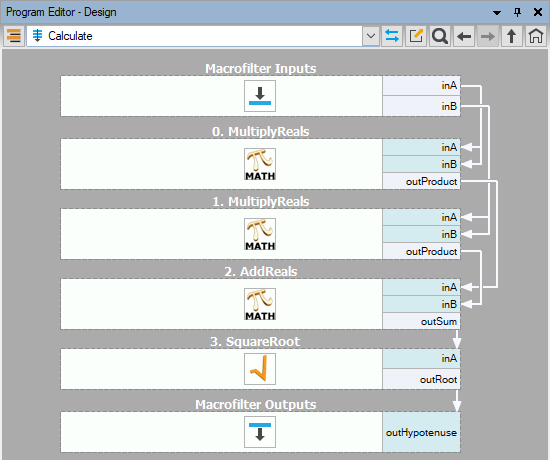Calculations using standard filters.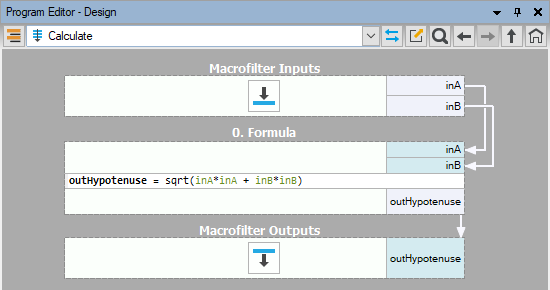Calculations using formula blocks.

The second approach, using formula blocks, is the most recommended. Data flow programming is just not well suited for numerical calculations and standard formulas, which can be defined directly in formula blocks, are much easier to read and understand. You can also think of this feature as an ability to embed little spreadsheets into your machine vision algorithms.

## Creating Formula Blocks

To create a formula block:

1. Drag and drop the Formula tool from the Toolbox (Logic & Math section) to the Program Editor: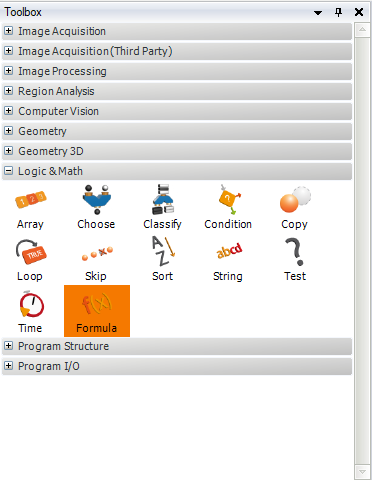2. Add inputs and outputs using the context menu or by clicking Add Input and Add Output links: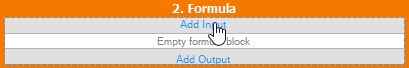3. For each input define the name and the type: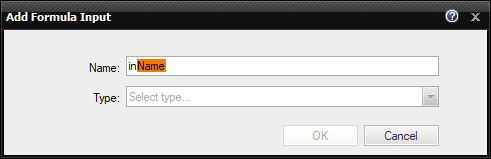4. In order to create an output, first press Add Output button. It will create a new output with template name: outName: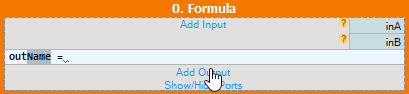Replace the highlighted Name with meaningful name. While typing a Tooltip with available options should appear: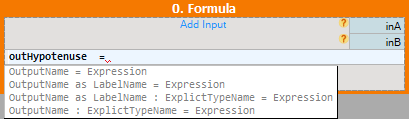Example usage of each option:

• After the name type equal sign = and the expression. There is no need to define a type of output. The type will be assigned automatically.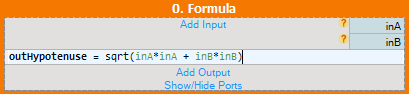• After the name add as followed by LabelName. This label will later appear as a formula output.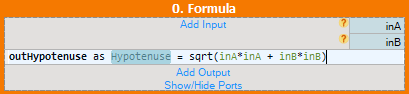• After the name add label as described in the previous point. Then add colon : and directly specify the output type: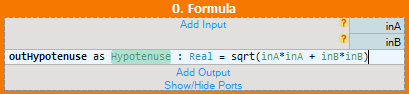• In the last option output type is defined directly. After the name add colon : and directly specify the output type: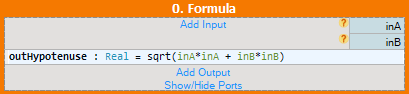Final formula should look like this: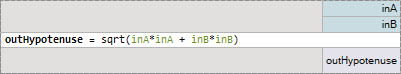Without output label.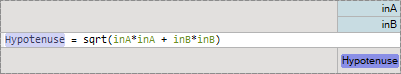With output label.
Not only the output data of the formulas can be labeled but also inputs and outputs of other filters outside of the formula. This way you can perform a direct call to another labeled data inside a formula without explicitly defining new formula inputs. In the image below the input A of the macrofilter is labeled (highlighted in violet color) and a direct call of this label (A) is performed inside the formula block. Later the output Hypotenuse is connected via a label with the macrofilter output, instead of linking it directly using an outgoing arrow connection.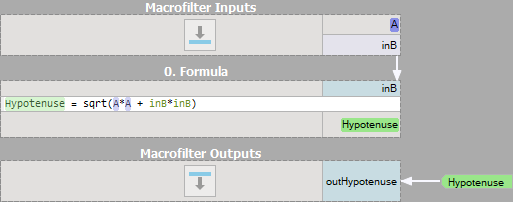Remarks

• Existing formulas can also be edited directly in a formula block in the Program Editor.
• It is also possible to create new inputs and outputs by dragging and dropping connections onto a formula block.
• Formula blocks containing incorrect formulas are marked with red background. Programs containing such filters cannot be run.
• When defining a formula for an output it is possible to use other outputs, provided that they are defined earlier. The order can be changed through the outputs context menu.
• For efficiency reasons it is advisable not to use "heavy" objects in formulas, such as images or regions.

## Syntax and Semantics

For complete information about the syntax and semantics please refer to the Formulas article in the Programming Reference.

 Previous: Remote Access to the Runtime Application Next: Managing Projects with Project Explorer